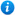# Comparing and Ordering Fractions - Year 3

Comparing and Ordering Fractions - Year 3: KS2 Maths Teaching Resource

Year 3 maths programme of study - Number - fractions:

• compare and order unit fractions, and fractions with the same denominators
• solve problems that involve all of the above

In this KS2 maths teaching resource pupils begin to compare and order unit fractions, fractions with like denominators and fractions with like numerators. It is an ideal resource to use in a lesson covering the curriculum objectives listed above and is suitable for mixed abilities.

This 57 slide PowerPoint presentation and 14 worksheets includes:

• What are fractions recap
• How to compare and order fractions with like denominators
• Comparing fractions with the same denominators activity and worksheets
• How to compare and order fractions with like numerators
• Comparing fractions with the same numerators activity and worksheets
• Ordering fractions on a number line activity and worksheet
• Ordering fractions activity and worksheet
• Comparing fractions true or false activity and worksheet
• Fraction word problems activity and worksheet
• Link to a comparing fractions game
• 3 further worksheets
'Comparing and Ordering Fractions - Year 3' is fully editable so that teachers are able to adapt the resource to suit the needs of each class they teach.
Preview 'Comparing and Ordering Fractions - Year 3' in full by clicking on the PowerPoint images above.

Our Price : £3.99 / 4 Credits## Get this resource as part of a bundle and save up to 58%## Year 3 Fractions Bundle

£7.99

By continuing to use the site, you agree to the use of cookies. You can change this and find out more by following this link